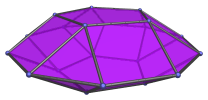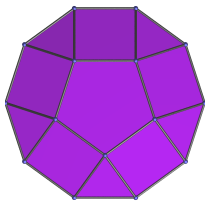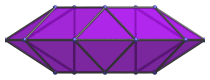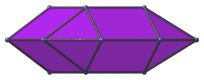# The Pentagonal Orthobicupola

The pentagonal orthobicupola is the 30th Johnson solid (J30). It has 20 vertices, 40 edges, and 22 faces (10 equilateral triangles, 10 squares, and 2 pentagons).The pentagonal orthobicupola can be constructed by joining two pentagonal cupolae to each other at their decagonal face, such that the square faces of each cupola touch the square faces of the other at the edges. The ortho in the name refers to how the orientation of the two cupolae are aligned with each other. Joining the two cupolae in gyro orientation produces the pentagonal gyrobicupola (J31) instead.

A decagonal prism can be inserted between the two constituent cupolae to make an elongated pentagonal orthobicupola (J38).

## Projections

Here are some views of the pentagonal orthobicupola from various angles:

Projection DescriptionTop view.Front view.Side view.

## Coordinates

The Cartesian coordinates of the pentagonal orthobicupola with edge length 2 are:

• (0, √((10+2√5)/5), ±2√((3−φ)/5))
• (±φ, √((5−√5)/10), ±2√((3−φ)/5))
• (±1, −√((5+2√5)/5), ±2√((3−φ)/5))
• (±2φ, 0, 0)
• (±1, ±√(3+4φ), 0)
• (±φ2, ±√(2+φ), 0)

where φ = (1+√5)/2 is the Golden Ratio, approximately 1.61803.

Last updated 17 May 2018.